Courses

# Fluid Mechanics 2

## 20 Questions MCQ Test Mock test series of SSC JE Civil Engineering | Fluid Mechanics 2

Description
This mock test of Fluid Mechanics 2 for Civil Engineering (CE) helps you for every Civil Engineering (CE) entrance exam. This contains 20 Multiple Choice Questions for Civil Engineering (CE) Fluid Mechanics 2 (mcq) to study with solutions a complete question bank. The solved questions answers in this Fluid Mechanics 2 quiz give you a good mix of easy questions and tough questions. Civil Engineering (CE) students definitely take this Fluid Mechanics 2 exercise for a better result in the exam. You can find other Fluid Mechanics 2 extra questions, long questions & short questions for Civil Engineering (CE) on EduRev as well by searching above.
QUESTION: 1

### The correct statement about ideal fluid is:

Solution:

A fluid is said to be ideal if it assumed to be both incompressible and non-viscous, its bulk modulus is infinite. An ideal fluid is a fluid that has several properties including the fact that it is:

Incompressible - the density is constant

Non-viscous - (Inviscid) fluid has no internal friction.

QUESTION: 2

### Specific gravity of water is 1.0 which is reported at a temperature of:

Solution:

Specific gravity of water is 1.0 which is reported at a temperature of 4°C. Specific gravity is a measure of density of liquid.

Some interesting facts about Specific gravity:

Specific gravity of water is actually a density of water in gram per cubic centimetre.

QUESTION: 3

### Quick sand comes under:

Solution:

Newtonian Fluids: Air, water, mercury, glycerine, kerosene and other engineering fluids under normal circumstances.

Pseudoplastic: Fine particle suspension, gelatine, blood, milk, paper pulp, polymeric solutions such as rubbers, paints.

Dilatant fluids: Ultra fine irregular particle suspension, sugar in water, aqueous suspension of rice starch, quicksand, butter printing ink.

Ideal plastics or Bingham fluids: Sewage sludge, drilling muds.

Viscoelastic fluids: Liquids solid combination in pipe flow, bitumen, tar, asphalt, polymerized fluids with drag reduction features.

Thixotropic: Printer’s ink, crude oil, lipstick, certain paints and enamels.

Rheopectic fluids: Very rare liquid - solid suspensions, gypsum suspension in water and bentonite solutions.

QUESTION: 4

Discharge Q in a triangular weir varies as:-

Solution:

Discharge through a triangular notch/weir is given by: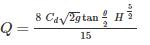Where,

H = height of liquid above apex of the notch

θ = Angle of notch

Cd = Coefficient of discharge

QUESTION: 5

For a uniform flow with depth of 0.6 m and Froude number of 2.0 in a rectangular channel, the specific energy will be -

Solution:

Froude number=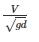Where,

V = velocity of flow through the rectangular channel

D = depth of flow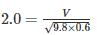V=4.85m/s

Specific Energy (E) is given by =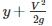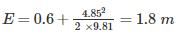QUESTION: 6

The major loss of hydraulic energy in pipe flow occurs in long pipe due to:-

Solution:

There are generally two types of losses occur in a pipe flow problem:

a) Major loss: Major head loss occurs due to friction, which is given by: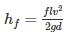Where f = friction factor=1. Sudden enlargement

2. Sudden contraction

3. Due to pipe bends

4. head loss at the entrance and exit of pipe etc.

QUESTION: 7

The eddy viscosity for turbulent flow is

Solution:

1. Flows become turbulent due to differences in velocity inside them, and the flow resistance increases. This resistive force acting on flows due to turbulence is called the Reynolds stress. This is an imaginary force that appears when flow fields are seen with coarse graining by time-averaging.

2. Viscosity, the proportionality constant of viscous force, is a physical quantity dependent on the type of material, while eddy viscosity is a physical quantity dependent on the state of flow.

QUESTION: 8

For a vacuum pressure of 4.5 m of water, the equivalent absolute pressure is:

Solution:

Absolute pressure = gauge pressure + local atmospheric pressure

-ve gauge pressure = vacuum pressure = 4.5 m of water

Local atmospheric pressure of water = 10.3 m of water

Equivalent absolute pressure = -4.5 + 10.33 = 5.83 m of water

QUESTION: 9

In a lock gate, the reaction between two gates is

Solution:

The lock gates are provided in navigation chambers to change a water level in a canal or river in navigation. There are two sets of gates, one set on either side of the chamber.

In a lock gate, the reaction between the two gates (R) is given by: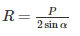P = Resultant water pressure on the lock gate

α = Inclination of the gate with the normal to the side of the lock.

QUESTION: 10

An orifice is said to be large, if

Solution:

An orifice is a small aperture through which the fluid passes. The thickness of an orifice in the direction of flow is very small in comparison to its other dimensions. An orifice is said to be large, if the available head of liquid is less than 5 times the height of orifice.

QUESTION: 11

In order, to avoid separation in Venturi meter the angle of divergence is kept

Solution: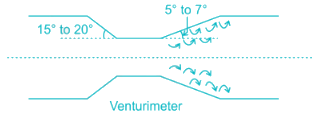In the diverging portion there is a decrease in velocity & subsequent increase in pressure. If divergence angle is very large, then back pressure will increase by great extent & eddies formation will take place resulting in flow separation. Thus, to avoid flow separation the divergence angle must not exceed more than 7° and range should be around 5 - 7°.

QUESTION: 12

The specific speed of a pump is defined as the speed of a unit of such a size that it discharge:

Solution:

The specific speed of a pump is defined as speed at which pump generates unit discharge under unit head.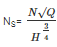where Q=discharge, H=head, Ns= specific speed, N= speed of pump.

QUESTION: 13

The discharge over a sharp - edged rectangular notch of width w depth h is equal to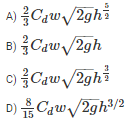Solution: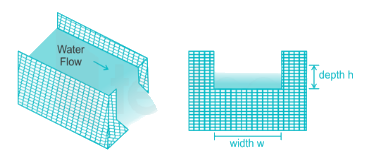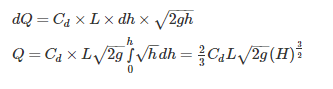QUESTION: 14

Froude number for weak and strong jump respectively are

Solution: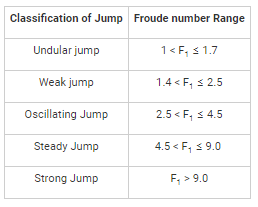QUESTION: 15

The point at which the resultant pressure on a immersed surface acts, is known as:

Solution:

Centre of pressure:

The point of action of total hydrostatic force on a submerged surface is called the centre of pressure. It is the point at which the resultant pressure on a immersed surface acts.

QUESTION: 16

The sequent depth ratio in a hydraulic jump termed in a horizontal rectangular channel is 5. The Froude number of the supercritical stream is

Solution: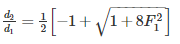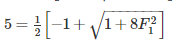F= 3.87

QUESTION: 17

In a laminar flow in a pipe the shear stress is:

Solution: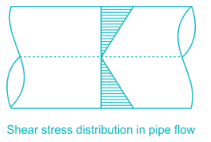QUESTION: 18

The flow of water in a wash hand basin when it is being emptied through a central opening, is an example of

Solution:

In a free vortex flow total mechanical energy remains constant. There is neither any energy interaction between an outside source and the flow, nor is there any dissipation of mechanical energy within the flow. The fluid rotates by virtue of some rotation previously imparted to it or because of some internal action.

Some examples are a whirlpool in a river, the rotatory flow that often arises in a shallow vessel when liquid flows out through a hole in the bottom (when water flows out from a bathtub or a wash basin), and flow in a centrifugal pump case just outside the impeller.

QUESTION: 19

A compound pipe of diameters d1, d2 and d3 having length l1, l2 and l3 respectively is to be replaced by an equivalent pipe of uniform diameter d and length l. The size of the equivalent pipe is given by

Solution:

For pipes in series equivalent pipe is given by: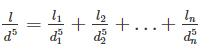For pipes in parallel equivalent pipe is given by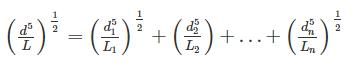QUESTION: 20

The equation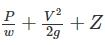Zconstant is based on the following assumptions regarding the flow of fluid

Solution:

The equation is Bernoulli’s equation. lt is based on following assumptions: